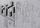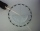# Sine - math word problems

#### Number of problems found: 155

• Resultant forceCalculate mathematically and graphically the resultant of a three forces with a common centre if: F1 = 50 kN α1 = 30° F2 = 40 kN α2 = 45° F3 = 40 kN α3 = 25°
• Triangle SASCalculate the triangle area and perimeter, if the two sides are 51 cm and 110 cm long and angle them clamped is 130 °.
• Inner anglesThe inner angles of the triangle are 30°, 45° and 105° and its longest side is 10 cm. Calculate the length of the shortest side, write the result in cm up to two decimal places.
• BearingA plane flew 50 km on a bearing 63°20' and the flew on a bearing 153°20' for 140km. Find the distance between the starting point and the ending point.
• Space diagonal anglesCalculate the angle between the body diagonal and the side edge c of the block with dimensions: a = 28cm, b = 45cm and c = 73cm. Then, find the angle between the body diagonal and the plane of the base ABCD.
• Triangle KLBIt is given equilateral triangle ABC. From point L which is the midpoint of the side BC of the triangle it is drwn perpendicular to the side AB. Intersection of perpendicular and the side AB is point K. How many % of the area of the triangle ABC is area o
• SSA and geometryThe distance between the points P and Q was 356 m measured in the terrain. The PQ line can be seen from the viewer at a viewing angle of 107° 22 '. The observer's distance from P is 271 m. Determine the viewing angle of P and observer.
• Paper boxCalculate the consumption of paper on the box-shaped quadrangular prism with rhombic footstall, base edge a=6 cm and the adjacent base edges forms an angle alpha = 60 °. Box height is 10 cm. How many m2 of the paper consumed 100 such boxes?
• SailboatThe 20 m long sailboat has an 8 m high mast in the middle of the deck. The top of the mast is fixed to the bow and stern with a steel cable. Determine how much cable is needed to secure the mast and what angle the cable will make with the ship's deck.
• Two forcesThe two forces F1 = 580N and F2 = 630N, have an angle of 59 degrees. Calculate their resultant force, F.What is the volume of a quadrilateral oblique prism with base edges of length a = 1m, b = 1.1m, c = 1.2m, d = 0.7m, if a side edge of length h = 3.9m has a deviation from the base of 20° 35 ´ and the edges a, b form an angle of 50.5°.
• Water channelThe cross section of the water channel is a trapezoid. The width of the bottom is 19.7 m, the water surface width is 28.5 m, the side walls have a slope of 67°30' and 61°15'. Calculate how much water flows through the channel in 5 minutes if the water flo
• Goat and circlesWhat is the radius of a circle centered on the other circle and the intersection of the two circles is equal to half the area of the first circle? This task is the mathematical expression of the role of agriculture. The farmer has circular land on which g
• Black buildingKeith built building with a rectangular shape 6.5 m × 3.9 m. Calculate how much percent exceeded the limit 25 m2 for small building. Building not built in accordance with the law is called "black building". Calculate the angle that the walls were clenchi
• Refractive indexThe light passes through the interface between air and glass with a refractive index of 1.5. Find: (a) the angle of refraction if light strikes the interface from the air at an angle of 40°. (b) the angle of refraction when light strikes the glass interfa

Do you have an interesting mathematical word problem that you can't solve it? Submit a math problem, and we can try to solve it.

We will send a solution to your e-mail address. Solved examples are also published here. Please enter the e-mail correctly and check whether you don't have a full mailbox.

Please do not submit problems from current active competitions such as Mathematical Olympiad, correspondence seminars etc...

Most natural application of trigonometry and trigonometric functions is a calculation of the triangles. Common and less common calculations of different types of triangles offers our triangle calculator. Word trigonometry comes from Greek and literally means triangle calculation.